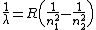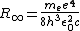# Rydberg constant

(redirected from Rydberg Frequency)
Also found in: Dictionary, Thesaurus.

## Rydberg constant

(rĭd`bərg), physical constant used in studies of the spectrumspectrum,
arrangement or display of light or other form of radiation separated according to wavelength, frequency, energy, or some other property. Beams of charged particles can be separated into a spectrum according to mass in a mass spectrometer (see mass spectrograph).
of a substance. Its value for hydrogen is 109,737.3 cm−1.

## Rydberg constant

The most accurately measured of the fundamental constants; it is a universal scaling factor for any spectroscopic transition and an important cornerstone in the determination of other constants.

This constant was introduced empirically. J. Balmer's formula described the visible spectral lines of atomic hydrogen, while J. Rydberg's formula applied to the spectra of many elements. Their results may be summarized by Eq. (1),

(1)where λ is the wavelength of the spectral line and R is a constant. In Balmer's account of the visible hydrogen spectrum, n1 was equal to 2, while n2 took on the integer values 3, 4, 5, and so forth. In Rydberg's more general work, n1 and n2 differed slightly from integer values. A remarkable result of Rydberg's work was that the constant R was the same for all spectral series he studied, regardless of the element. This constant R has come to be known as the Rydberg constant.

Applied to hydrogen, Niels Bohr's atomic model leads to Balmer's formula with a predicted value for the Rydberg constant given by Eq. (2),

(2)where me is the electron mass, e is the electron charge, h is Planck's constant, ε0 is the permittivity of vacuum, and c is the speed of light. The equation expresses the Rydberg constant in SI units. To express it in cgs units, the right-hand side must be multiplied by (4&pgr;ε0)2. The subscript ∞ means that this is the Rydberg constant corresponding to an infinitely massive nucleus.

E. Schrödinger's wave mechanics predicts the same energy levels as the simple Bohr model, but the relativistic quantum theory of P. A. M. Dirac introduces small corrections or fine-structure splittings. The modern theory of quantum electrodynamics predicts further corrections. Additional small hyperfine-structure corrections account for the interaction of the electron and nuclear magnetic moments. See Fine structure (spectral lines), Hyperfine structure

The Rydberg constant is determined by measuring the wavelength or frequency of a spectral line of a hydrogenlike atom or ion. The highest resolution and accuracy has been achieved by the method of Doppler-free two-photon spectroscopy, which permits the observation of very sharp resonance transitions between long-living states. The 2002 adjustment of the fundamental constants, taking into account different measurements, adopted the value R = 10,973,731.568,525 ± 0.000,073 m-1 for the Rydberg constant. The measurements provide an important cornerstone for fundamental tests of basic laws of physics. See Atomic structure and spectra, Fundamental constants, Laser, Laser spectroscopy

McGraw-Hill Concise Encyclopedia of Physics. © 2002 by The McGraw-Hill Companies, Inc.
The following article is from The Great Soviet Encyclopedia (1979). It might be outdated or ideologically biased.

## Rydberg Constant

(R), a physical constant introduced by J. R. Rydberg in 1890 in the study of atomic spectra.

The Rydberg constant is found in the expressions for energy levels and emission frequencies of atoms. If the mass of a nucleus of an atom is taken as infinitely great relative to the mass of an electron (the nucleus is stationary), then, according to quantum-mechanical calculation, R = 2π2me4/ch3 = 10,973,731.77 ± 0.83 m-1 (as of 1976), where e and m are the charge and mass of an electron, c is the velocity of light, and h is Planck’s constant, if the movement of the nucleus is taken into account, the mass of an electron is replaced by the reduced mass of the electron and nucleus, and then Ri = R/(1 + m/M), where M is the mass of the nucleus. For light atoms (hydrogen H, deuterium D, and helium 4He), the Rydberg constant has the following values (in m-1): RH = 10,967,759.3, RD = 10,970,741.7, and R4He = 10,972,226.7.

### REFERENCE

Taylor, B., W. Parker, and D. Langenberg. Fundamental’nye konstanty i kvantovaia elektrodinamika. Moscow, 1972. (Translated from English.)